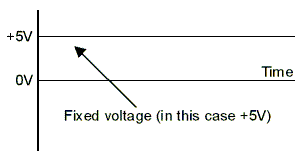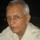Coffee Room
Discuss anything here - everything that you wish to discuss with fellow engineers.
12840 Members
Join this group to post and comment.Naman Agarwal • Aug 17, 2012

# Zero Frequency Of dc

how can we proove that the frequency of dc is zero???Abhishek Rawal • Aug 17, 2012
In Direct current, current/voltage doesn't change with time, hence frequency (f) = zero.Frequency = (number of changes/sec), in this case the number of times the voltage changes.
A pure DC is constant. So number of changes = 0.
0/secs = 0.
However if you have a DC with a wave form such as square wave, saw tooth or even a single phase full wave rectified unfiltered DC there will be a frequency, which will be the numbers of peaks/sec.Jeffrey Arulraj • Oct 14, 2012
to experimentally verify this use a circuit having a source and capacitor in series to it

Measure the voltage across the capacitor if it varies then the source is AC but if it is stable at the input voltage it is DC supply